# ML Aggarwal Solutions for Class 7 Maths Chapter 15 Visualising Solid Shapes

ML Aggarwal Solutions for Class 7 Maths Chapter 15 Visualising Solid Shapes are available here, and it contains solutions for the exercise given in the textbook. These Solutions are explained in a proper step-by-step format in a simple language by expert tutors. We suggest students practice ML Aggarwal Solutions for Class 7 Maths Chapter 15 to get more marks in the exam.

Chapter 15 – Visualising Solid Shapes. Now, let us have a look at some of the concepts discussed in this chapter, Introduction to Plane Figures and Solid Figures, Faces, Edges and Vertices, Nets for Building 3-D Shapes, Drawing Solids on a Flat Surface, Oblique Sketches, Isometric Sketches Visualising Solid Objects.

## Download the PDF of ML Aggarwal Solutions for Class 7 Maths Chapter 15 Visualising Solid Shapes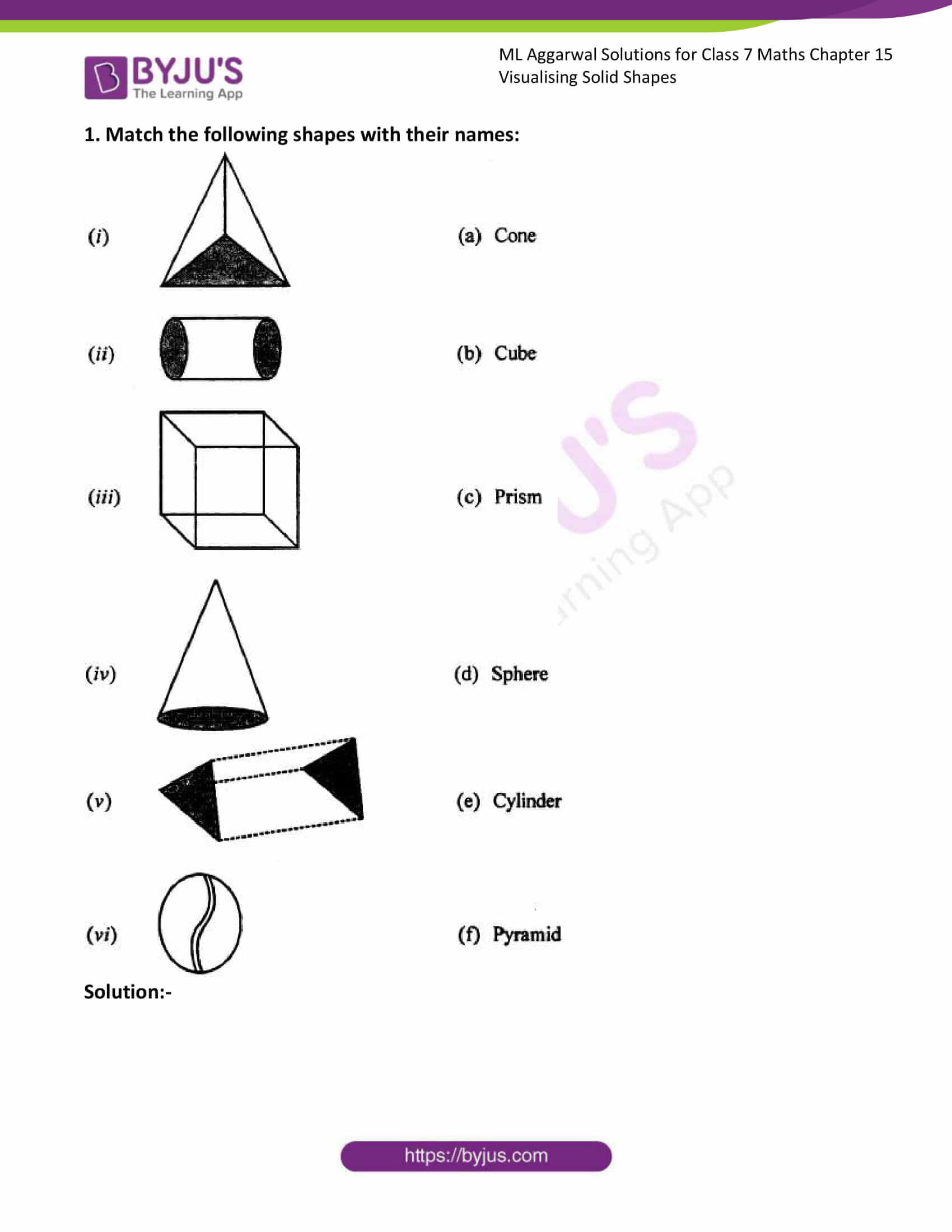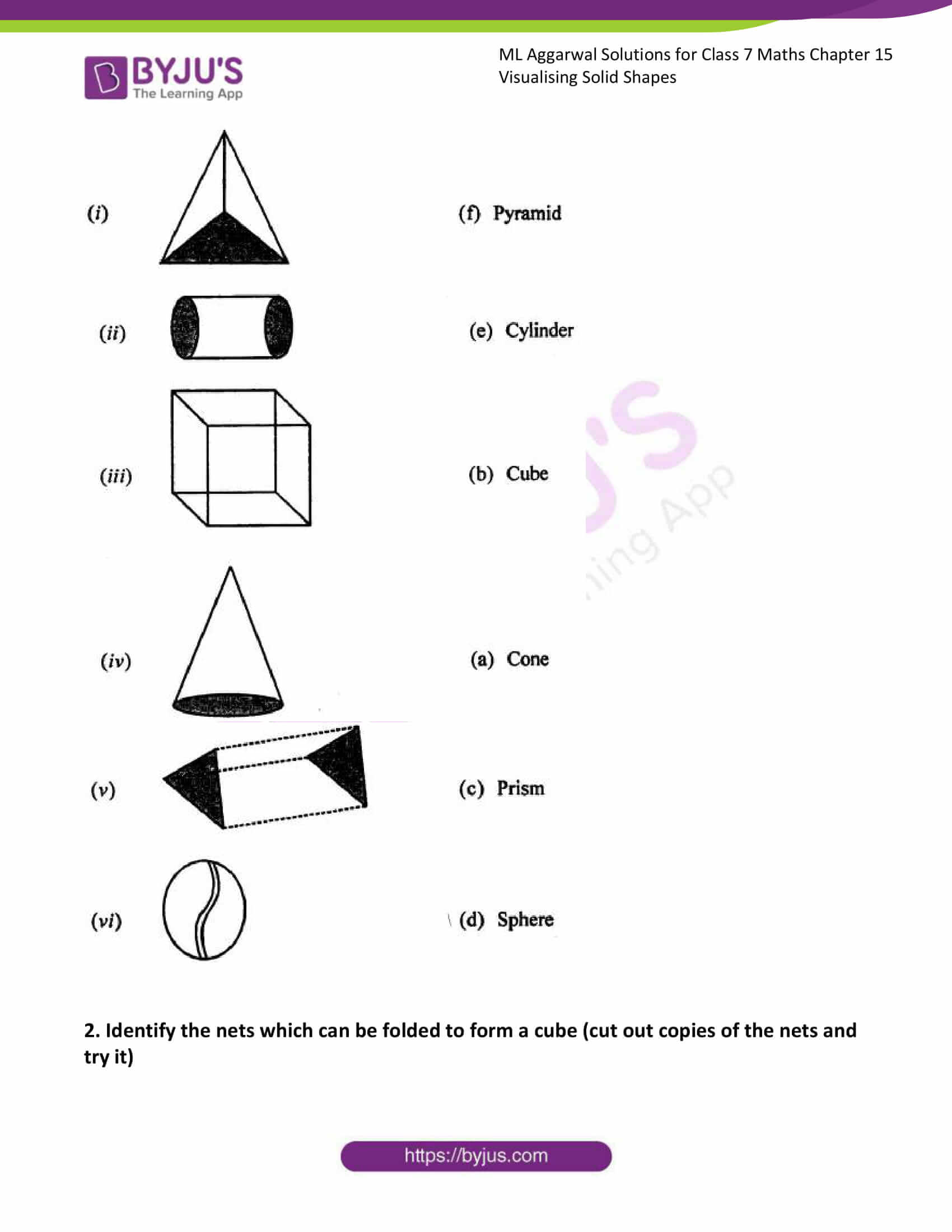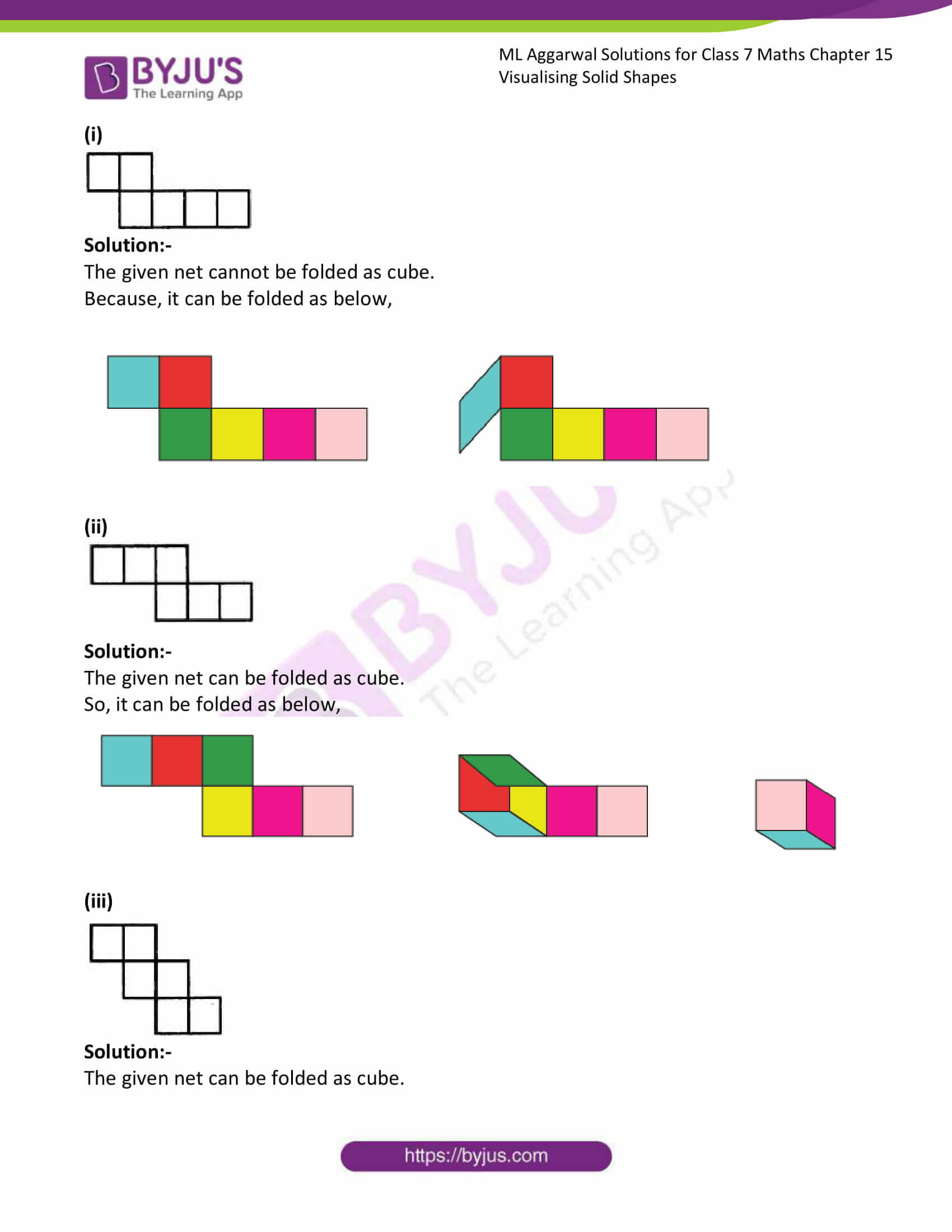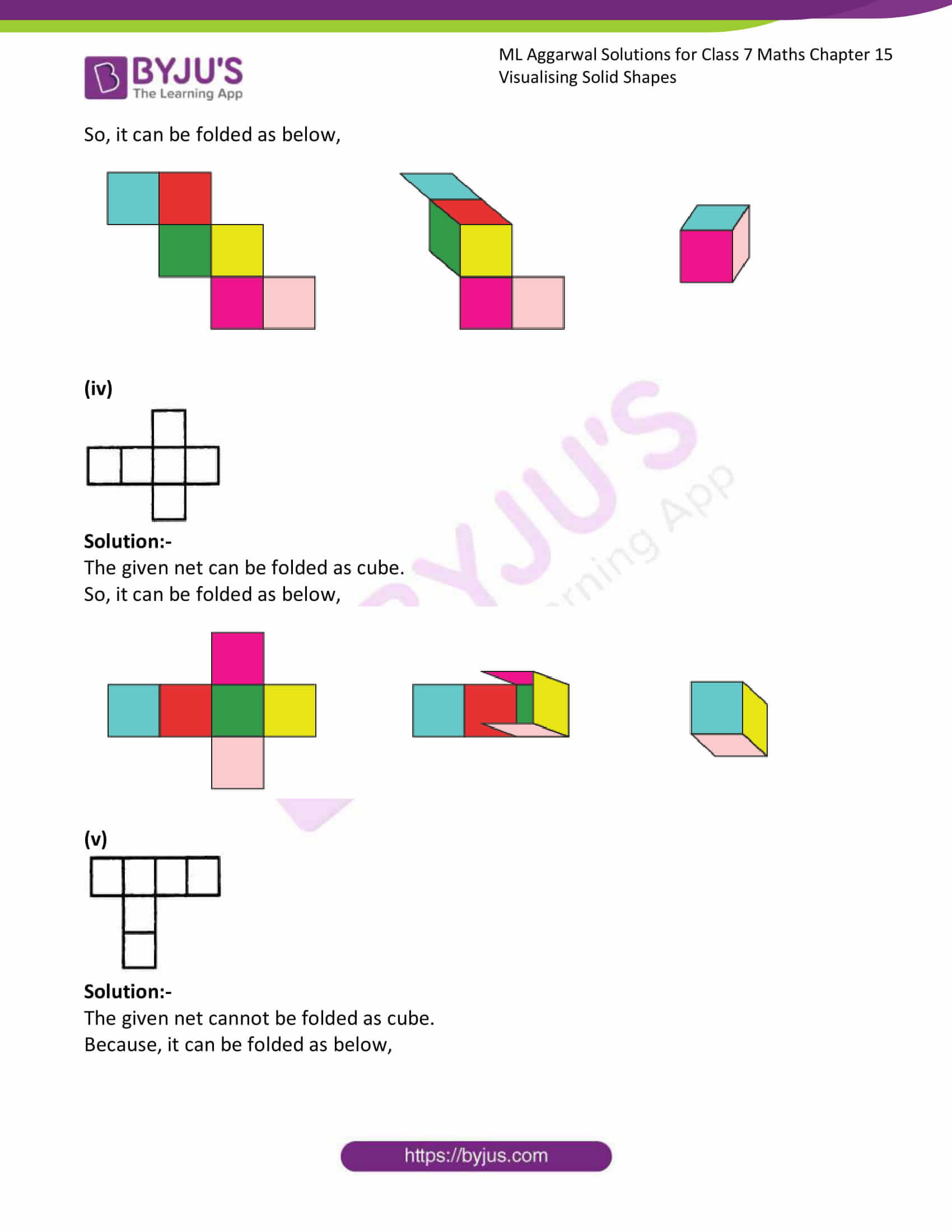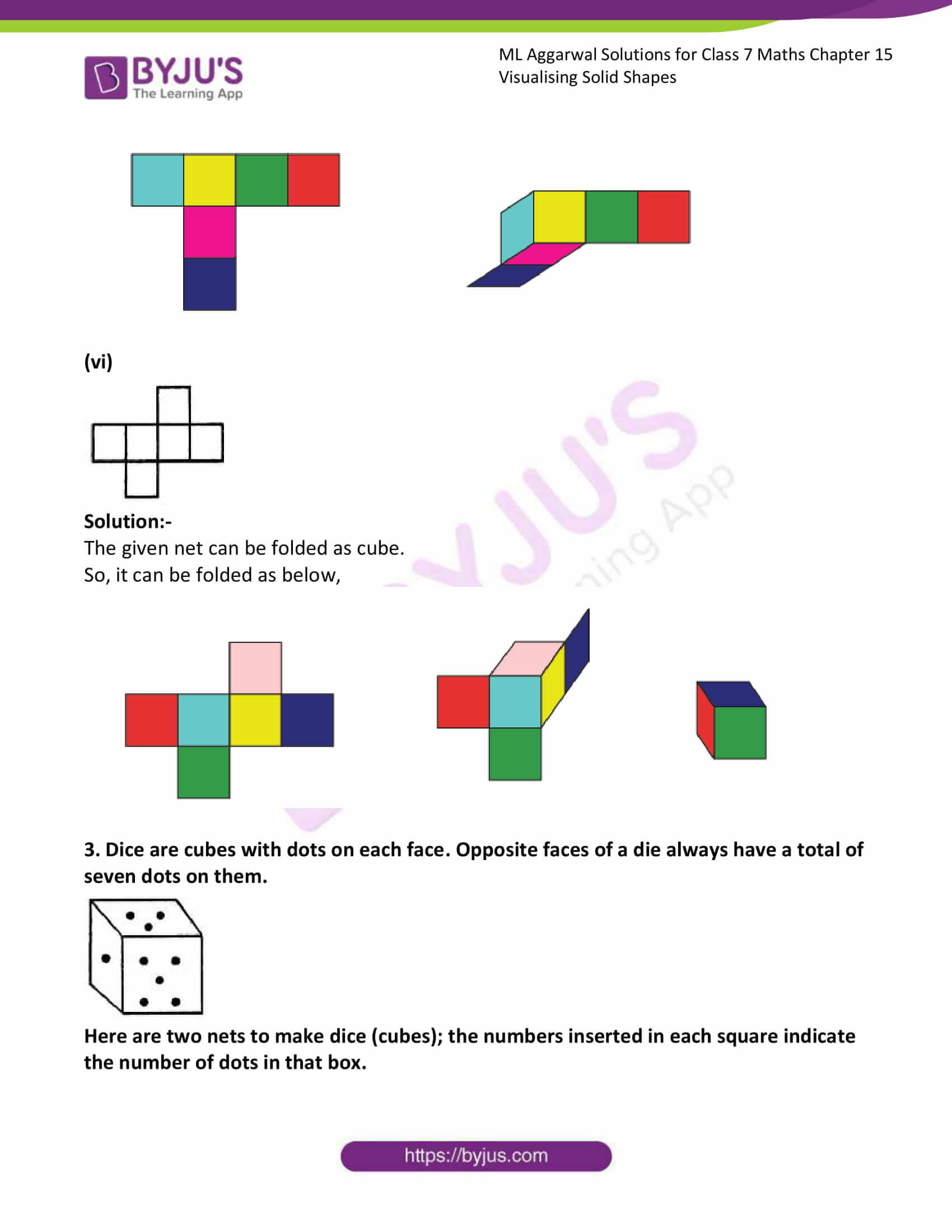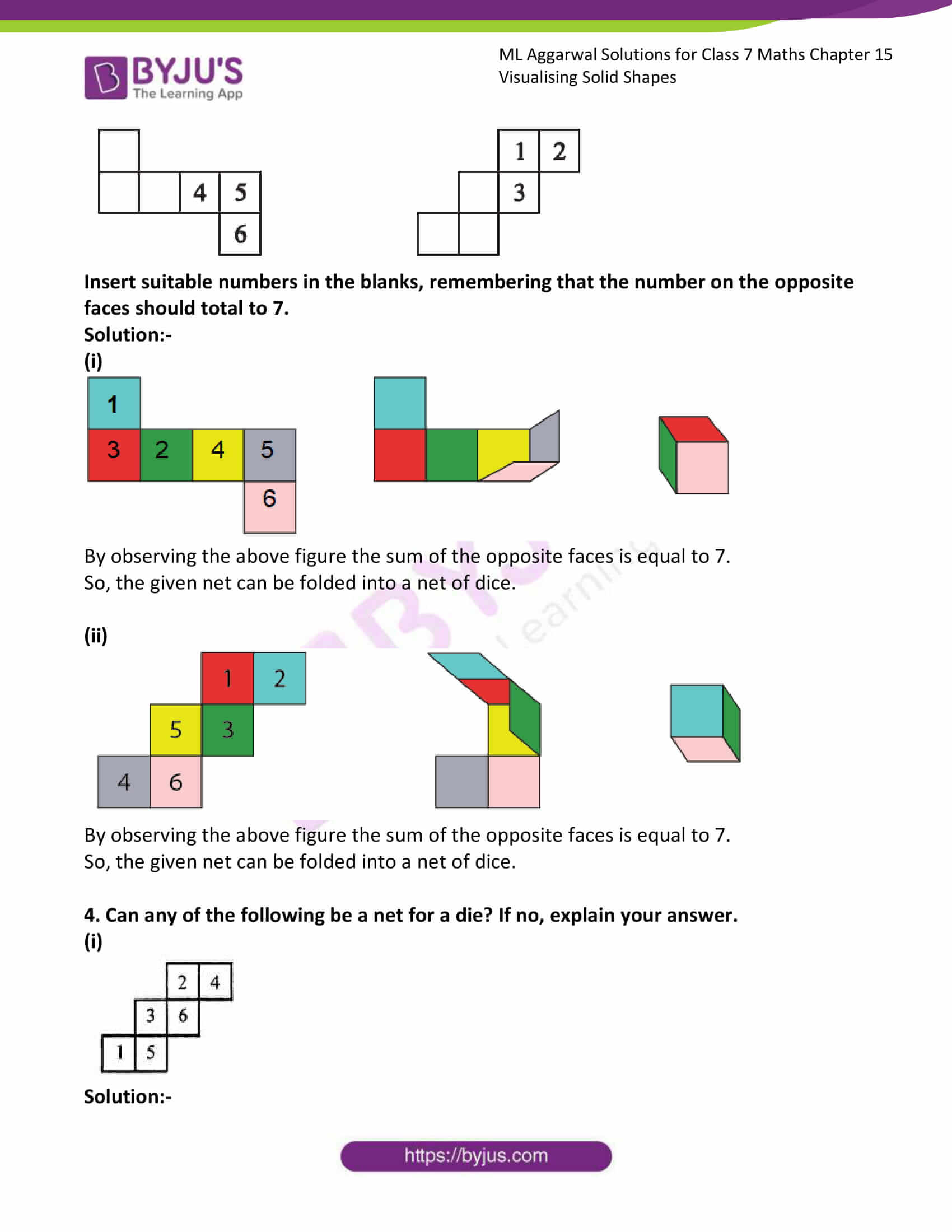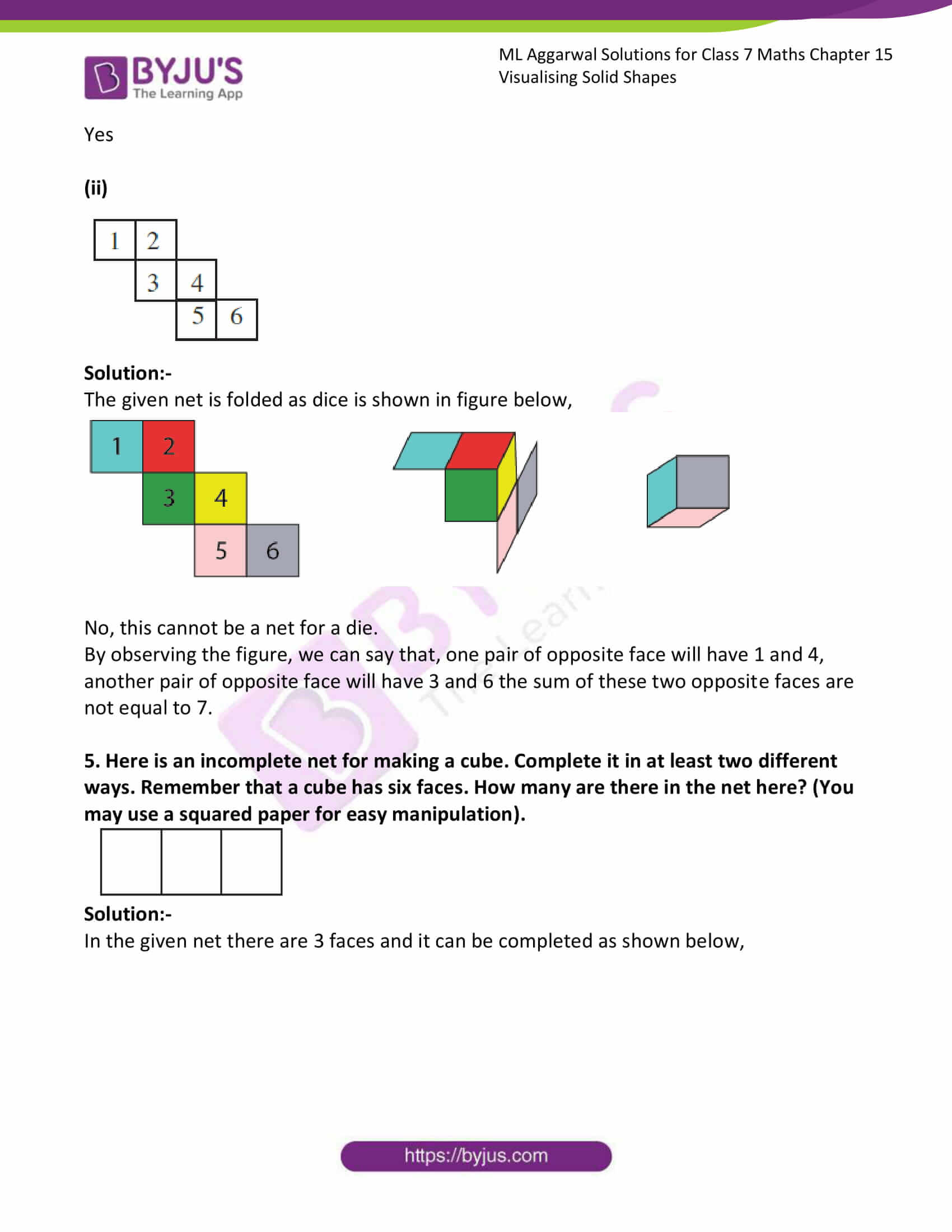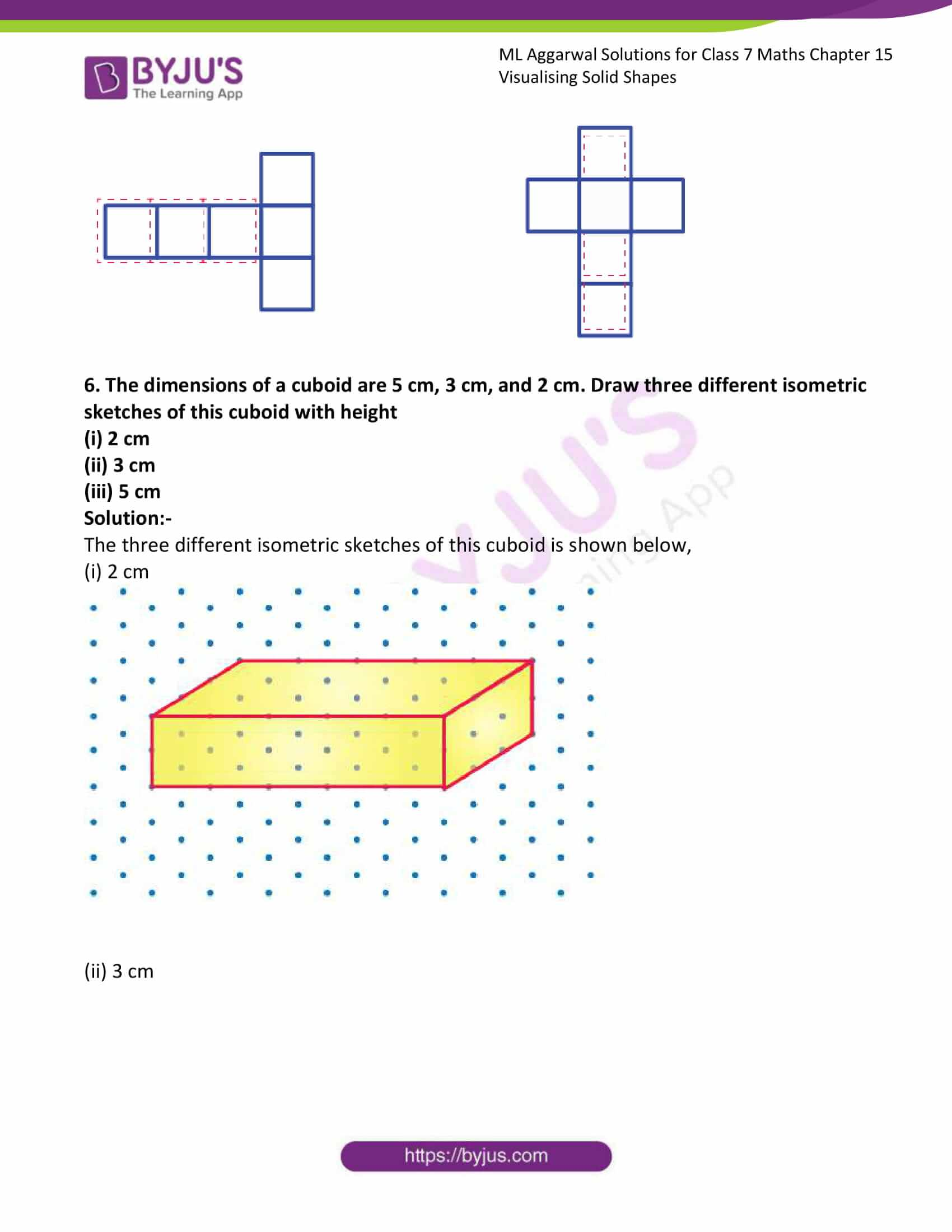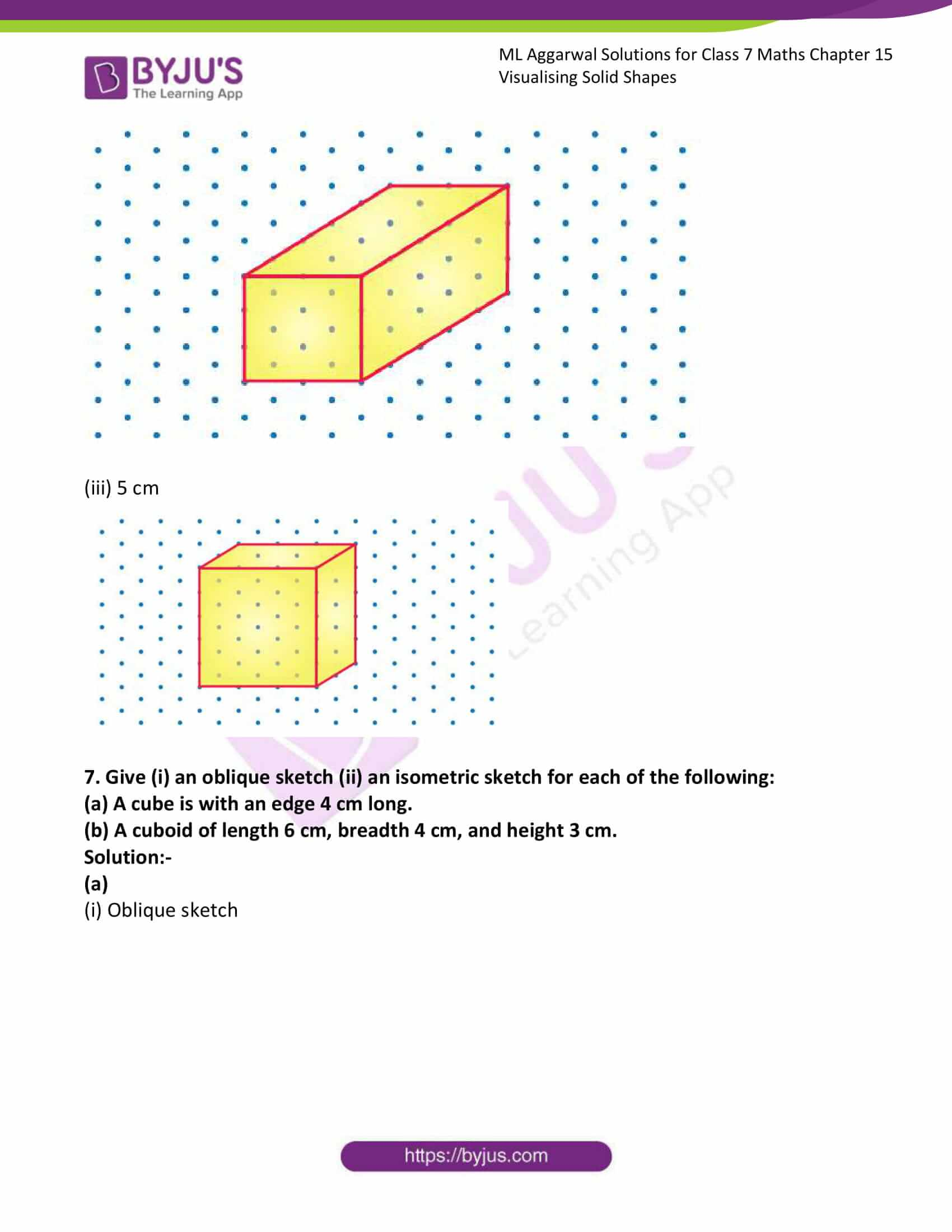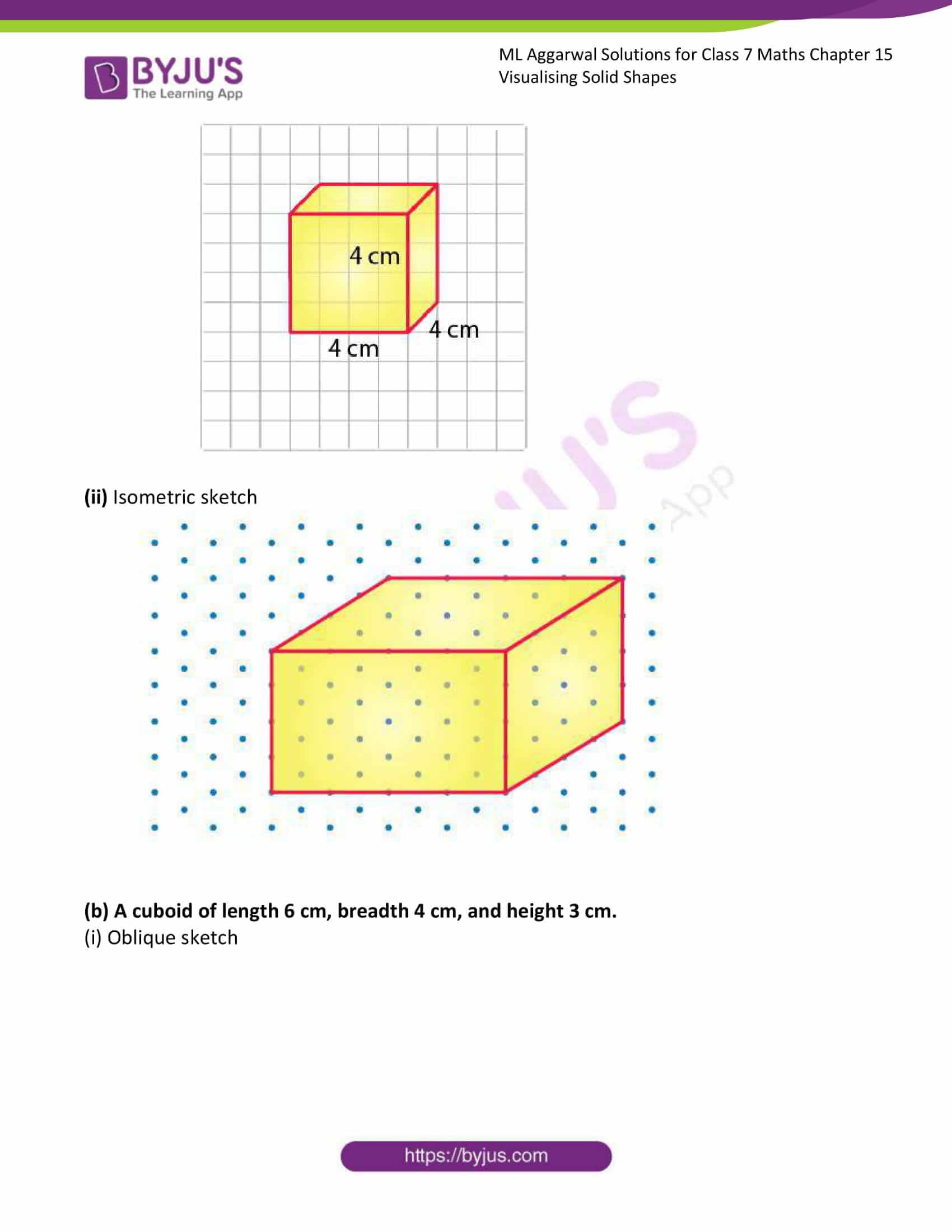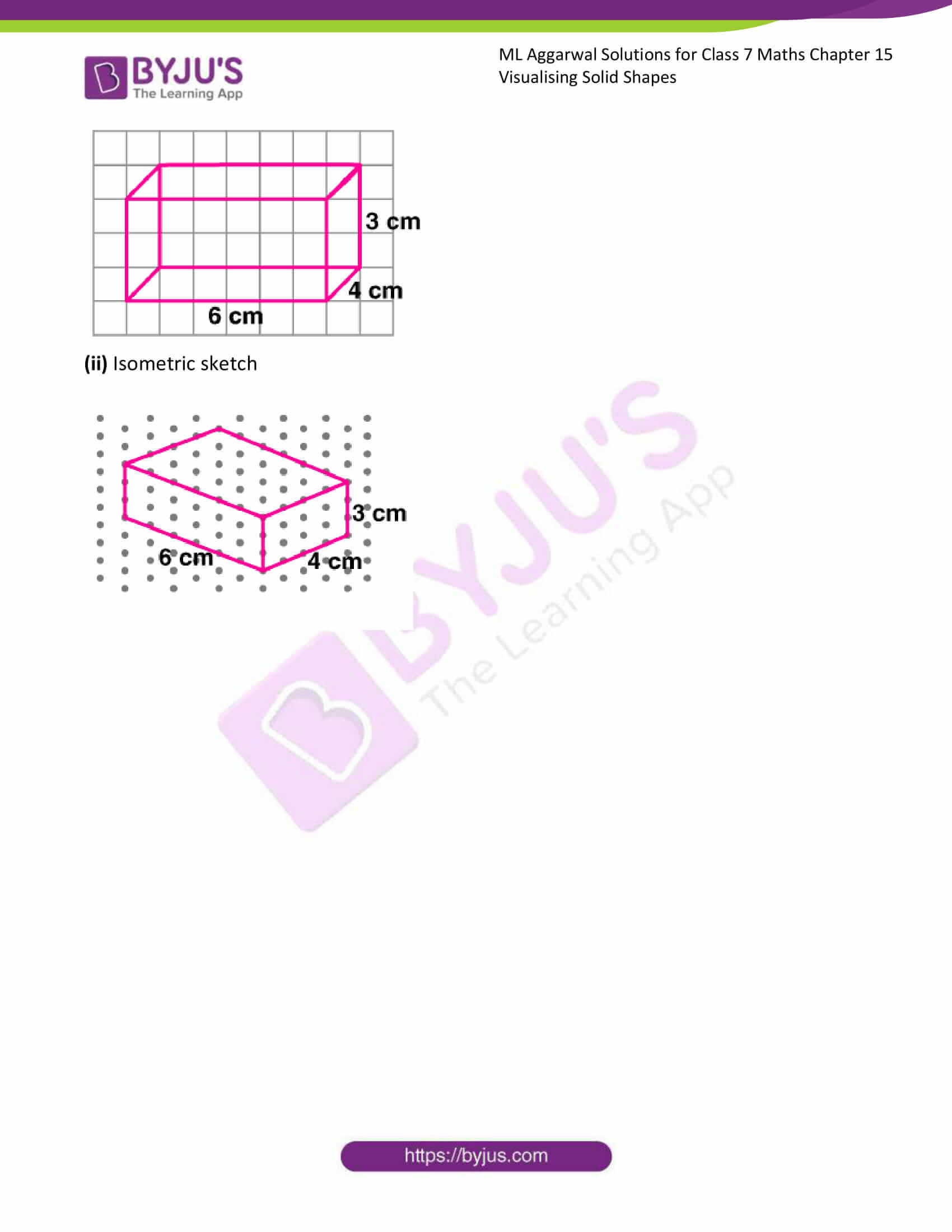### Access answers to ML Aggarwal Solutions for Class 7 Maths Chapter 15 Visualising Solid Shapes

1. Match the following shapes with their names: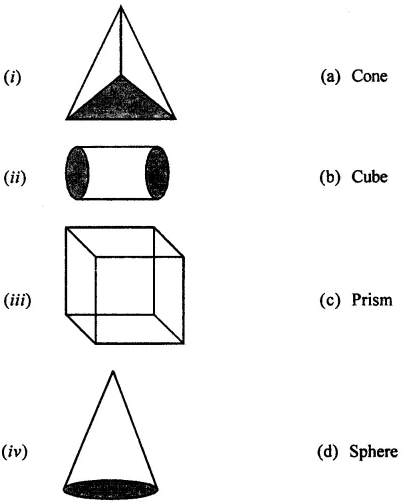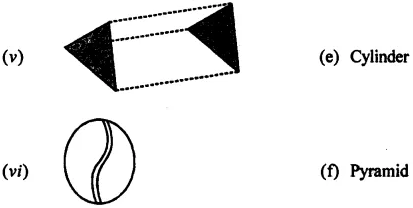Solution:-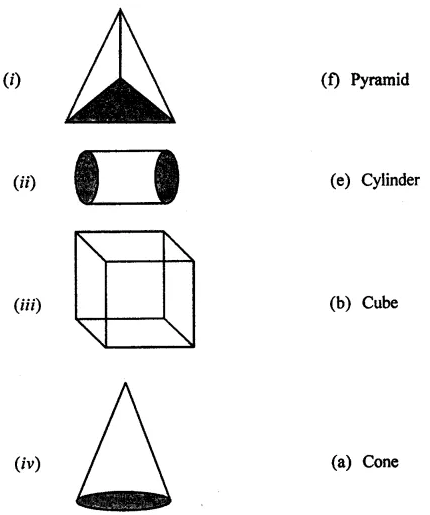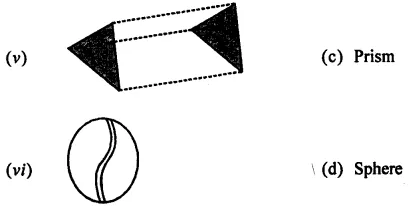2. Identify the nets which can be folded to form a cube (cut out copies of the nets and try it)

(i)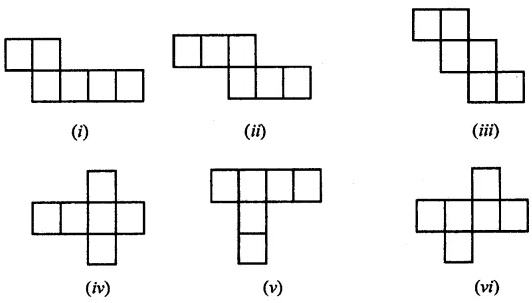Solution:-

The given net cannot be folded as cube.

Because, it can be folded as below,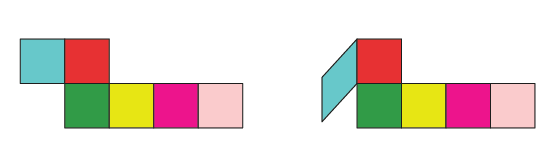(ii)Solution:-

The given net can be folded as cube.

So, it can be folded as below,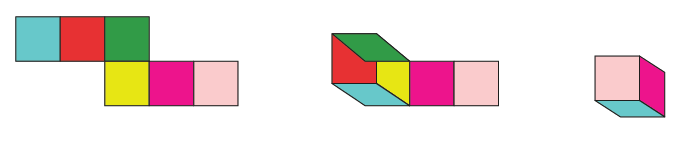(iii)Solution:-

The given net can be folded as cube.

So, it can be folded as below,(iv)Solution:-

The given net can be folded as cube.

So, it can be folded as below,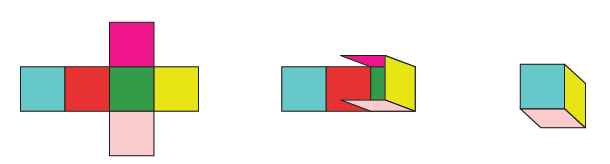(v)Solution:-

The given net cannot be folded as cube.

Because, it can be folded as below,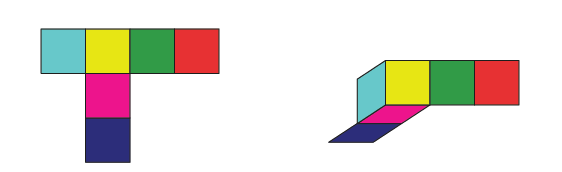(vi)Solution:-

The given net can be folded as cube.

So, it can be folded as below,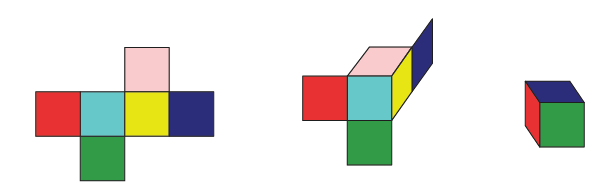3. Dice are cubes with dots on each face. Opposite faces of a die always have a total of seven dots on them.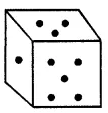Here are two nets to make dice (cubes); the numbers inserted in each square indicate the number of dots in that box.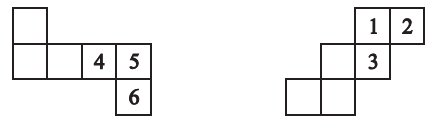Insert suitable numbers in the blanks, remembering that the number on the opposite faces should total to 7.

Solution:-

(i)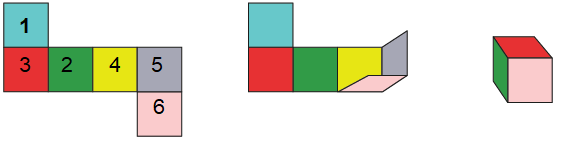By observing the above figure the sum of the opposite faces is equal to 7.

So, the given net can be folded into a net of dice.

(ii)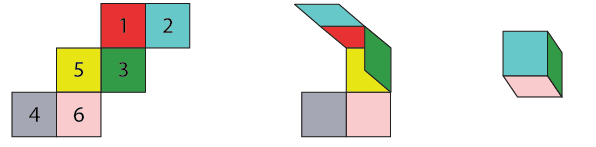By observing the above figure the sum of the opposite faces is equal to 7.

So, the given net can be folded into a net of dice.

4. Can any of the following be a net for a die? If no, explain your answer.

(i)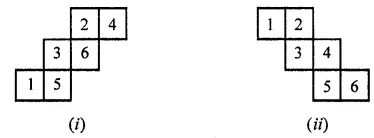Solution:-

Yes

(ii)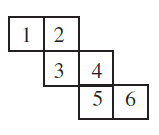Solution:-

The given net is folded as dice is shown in figure below,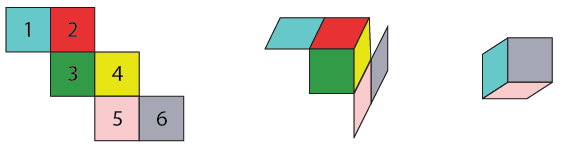No, this cannot be a net for a die.

By observing the figure, we can say that, one pair of opposite face will have 1 and 4, another pair of opposite face will have 3 and 6 the sum of these two opposite faces are not equal to 7.

5. Here is an incomplete net for making a cube. Complete it in at least two different ways. Remember that a cube has six faces. How many are there in the net here? (You may use a squared paper for easy manipulation).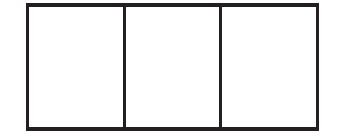Solution:-

In the given net there are 3 faces and it can be completed as shown below,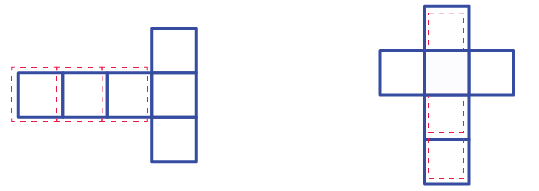6. The dimensions of a cuboid are 5 cm, 3 cm, and 2 cm. Draw three different isometric sketches of this cuboid with height
(i) 2 cm
(ii) 3 cm
(iii) 5 cm

Solution:-

The three different isometric sketches of this cuboid is shown below,

(i) 2 cm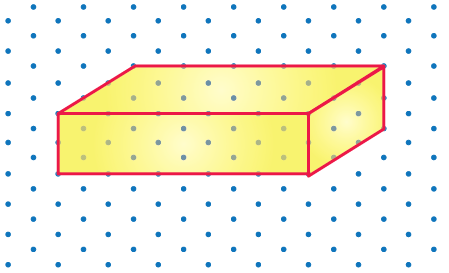(ii) 3 cm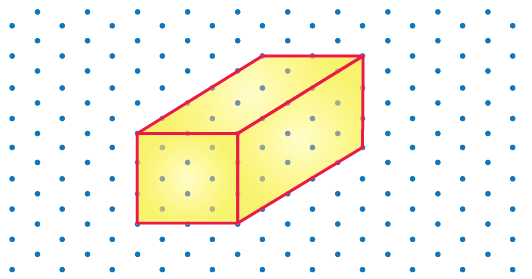(iii) 5 cm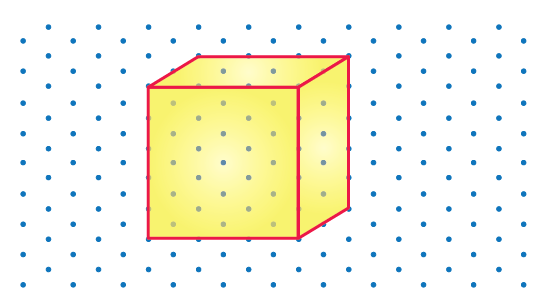7. Give (i) an oblique sketch (ii) an isometric sketch for each of the following:
(a) A cube is with an edge 4 cm long.
(b) A cuboid of length 6 cm, breadth 4 cm, and height 3 cm.

Solution:-

(a)

(i) Oblique sketch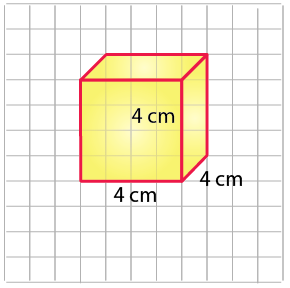(ii) Isometric sketch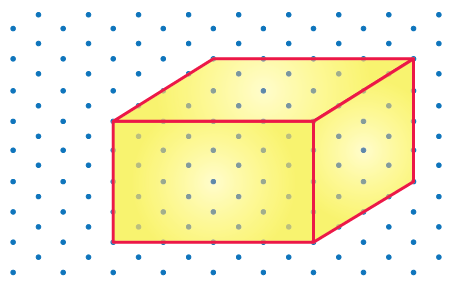(b) A cuboid of length 6 cm, breadth 4 cm, and height 3 cm.

(i) Oblique sketch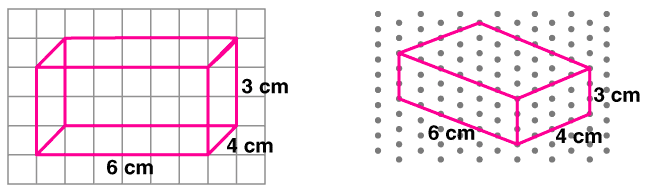(ii) Isometric sketch